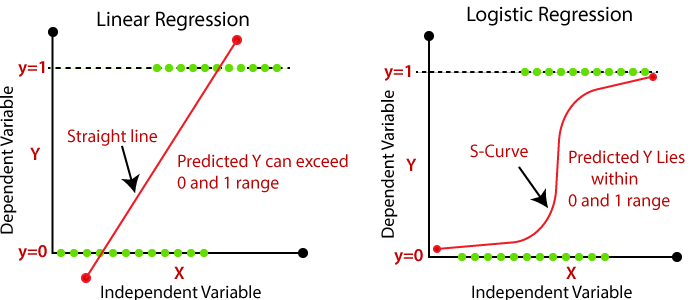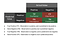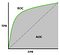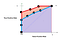# Logistic Regression

Logistic regression is a type of algorithm used for solving classification types of problems. Classification could be either binary such as 0/1, yes/no, High/low, male/female etc.. or multiple classes such as low/medium/ high, poor/average/excellent etc.,. It falls under the supervised learning algorithm, where for the given input features, output features/labels are also known. Unlike Linear regression which tries to find the relation between dependent(y) and independent variables(X) by establishing the best fit line, logistic regression also tries to regress a line that divides/separates dataset and classifies by using probabilistic functions.Linear Regression Vs Logistic Regression

Let us consider an example, depending on the average run rates secured by local cricketers in matches held that year, we are trying to select them, for the national team. So, there are only two possibilities, either the cricketer will be selected or rejected for the national team. It comes under binary classification and logistic regression could be used for solving this case.

Logistic regression classifies the dataset by drawing a linear separable hyperplane. In the case of binary classification, it says whether the dataset appears on this side or opposite of the hyperplane. Also, it says the probability value of the dataset belonging to either of the classes. For calculating the probability of dataset, logistic regression uses sigmoid function. The sigmoid function is a derivative function and its values always lie between 0 to 1.

Cost function:

The cost function in any algorithm is responsible for learning and reduce the error by adjusting the learnable parameters m and c. The cost function for Logistic regression is given by;

Cost function(J theta) = -1/m summation of [(y log p(x) + (1-y) log(1-p(x))]

where m is the total number of dataset

So, if the value of y is 0, then cost function becomes -log (1-p(x)) and if the value of y is 1, then cost function becomes -log p(x).

Evaluation of Logistic Regression:

The regression model uses R2 and Adjusted R2 statistics for evaluation of model accuracy. In case of classification model, accuracy of model is evaluated by using metrics such as accuracy, recall/sensitivity, precision, F1 score, specificity, AUC and ROC.

Let us first built a confusion matrix for determining the above accuracy metrics. confusion matrix components include True Positive (TP), True Negative (TN), False Positive (FP) and False Negative (FN).Confusion Matrix
1. Accuracy = (TP + TN) / (TP+TN+FP+FN)
2. Recall/Sensitivity/True positive rate= TP / TP + FN
3. Precision = TP / TP + FP
4. F1 Score = (2*Recall*Precision) / (Recall + Precision)
5. Specificity/True negative rate= TN / TN + FP
6. False positive rate = FP / FP + TN or (1-specificity)

ROC (Receiver Operating Characteristic)ROC Curve

The classification of entire data depends upon the threshold value we take. Say if the threshold value is 0.5, then dataset less than 0.5 falls under one class and dataset greater than or equal to 0.5 falls in another class. It is not always necessarily the threshold value to be 0.5 and it is important to pick threshold value correctly or the entire classification could be biased towards one class. ROC curve helps in sorting out this problem and enables us to pick the right threshold value using graphs. Roc is a graph drawn between True Positive Rate(recall) and False Positive Rate (1-specificity).

AUC (Area Under Curve):AUC Curve

Consider for a classification problem, we could use many algorithms to build a model such as Logistic regression, Decision tree, Random forest etc., AUC helps us to find out which algorithm suits best for the problem statement. The algorithm which covers more area is chosen as the best algorithm.